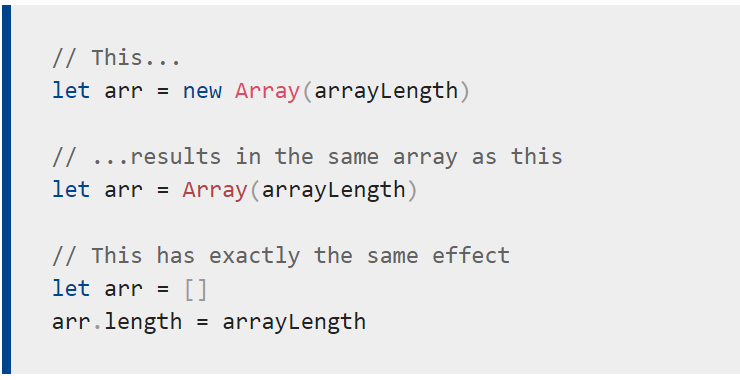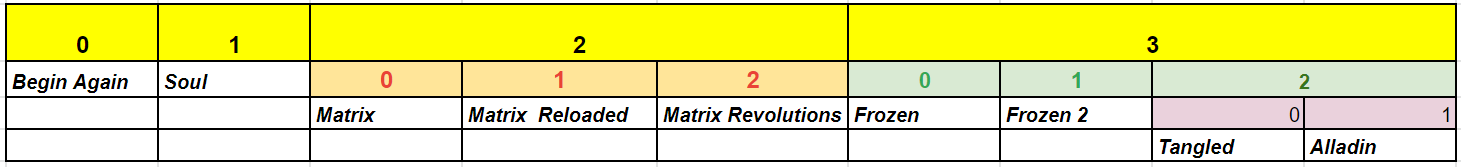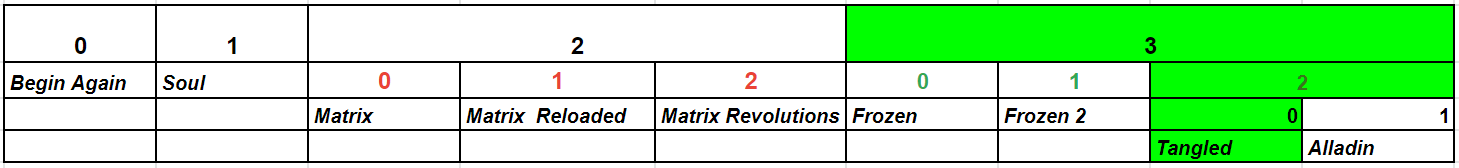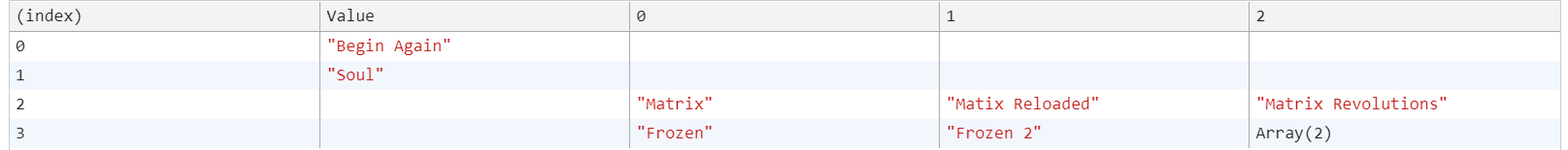# Understanding Nested Arrays in JavaScript

An array is an ordered collection of values: each value is called an element, and each element has a numeric position in the array, known as its index.

JavaScript lets us create arrays inside array called Nested Arrays. Nested Arrays have one or many arrays as the element of an array. This might be little confusing in definition but it is very interesting once we dig inside.## Creating a Nested Array

There are three syntax to create an array in JavaScript. Let's create nested arrays using those three methods to get an idea of Nested Arrays.

This one is just _equating _the variable to the array.

``````var favMovies = ['Begin Again', 'Soul', ['Matrix', 'Matix Reloaded', 'Matrix Revolutions'],['Frozen', 'Frozen 2', ['Tangled', 'Alladin']]]
``````

Second one is using the array method new Array().

And the last one is using the Array() which is similar to the new Array() .

Note that all these methods yield the same result. Now that we know how to create the nested arrays, let's see how to access the elements of the Nested Arrays.mn

## Understanding how indices is assigned to the elements

The arrays are listed according to the index. Below diagram explains how the indices are assigned to the elements in Nested Array.Say we want to access the value 'Tangled', we can navigate to it using this table.``````console.log(favMovies)
``````

Similarly we can access any element with the help of index.

Output from the console (console.table(favMovies))## Flatten the nested Array

There are ways to flatten the nested array. We can turn it into normal array by using the below methods.

### 1. Using the method Array.flat()

Array.flat() method produces a new array by concatenating all sub arrays recursively up to the depth you specify.

Simply put, if you have an array of arrays (maybe more arrays within them), flat() will help you to join all entries together into a single array.

### 2. Using Array.toString() and String.split() methods

We can convert the array to string and the split it using .split() method. That way we get the array.

### Conclusion

And there we have it! I hope you found this useful. Thanks!